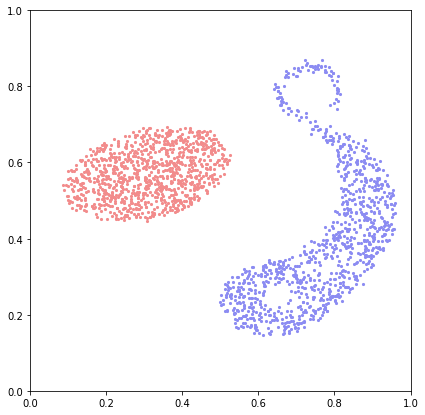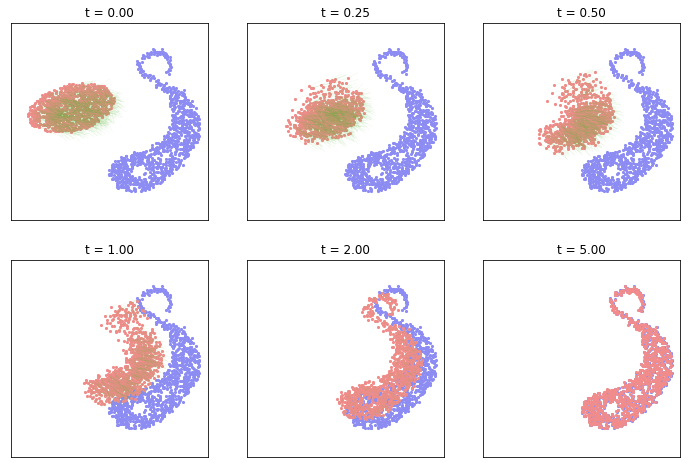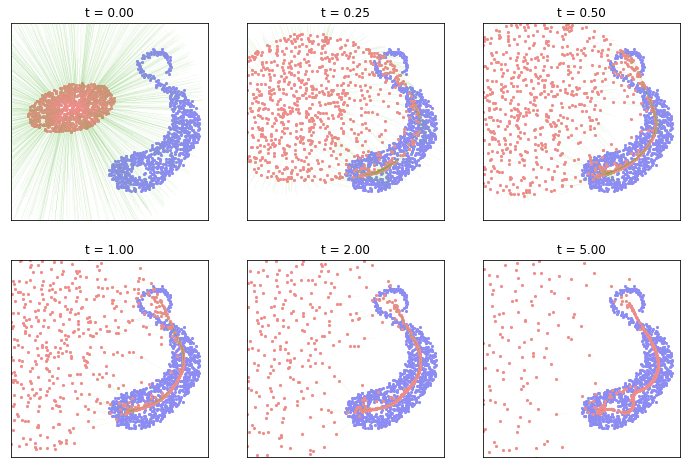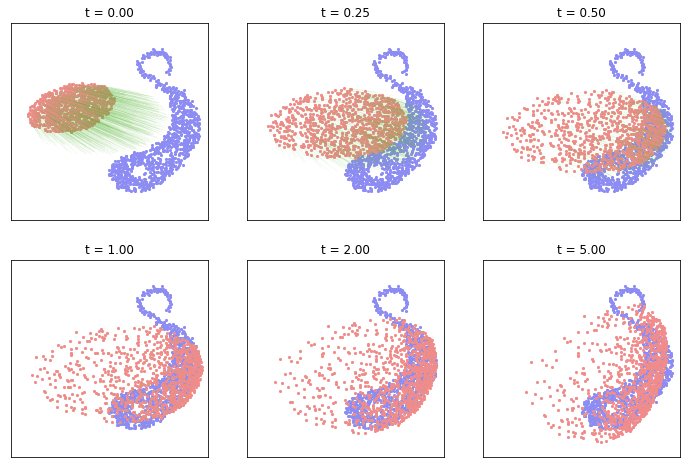# Gradient flows between sampled measures¶

(Author: Jean Feydy)

In this notebook, we showcase the properties of several geometric divergences defined on the space of probability measures: Kernel Norms (aka. Maximum Mean Discrepancies), Maximum Likelihoods of Gaussian Mixture Models (aka. sum-Hausdorff distances) and Optimal Transport costs (aka. Wasserstein or Earth-Mover's distances).

In :
# Import the standard array-related libraries (MATLAB-like)
import numpy as np
import matplotlib.pyplot as plt
import display # narrow jupyter column

%matplotlib inline

In :
# Import the automatic differentiation + GPU toolbox
import torch
use_cuda = torch.cuda.is_available() # Shall we use the GPU?
tensor   = torch.cuda.FloatTensor if use_cuda else torch.FloatTensor
dtype    = tensor

# Let's keep things fast by default. Feel free to increase!
NPOINTS = 1000 if use_cuda else 200
numpy = lambda x : x.detach().cpu().numpy()


To keep things simple and allow us to assess graphically the performances of our methods, we will work with measures $\alpha$ and $\beta$ sampled on the unit square:

\begin{align} \alpha &~=~ \sum_{i=1}^\text{N} \alpha_i\delta_{x_i}, & \beta &~=~ \sum_{j=1}^\text{M} \beta_j\delta_{y_j}, \end{align}

where $\alpha_i$, $\beta_j$ are positive weights associated to the samples $x_i$ and $y_j$ in $\mathbb{R}^2$. In this notebook, we will focus on the case where $\alpha$ and $\beta$ are probability measures:

\begin{align} \sum_{i=1}^\text{N} \alpha_i~=~1~=~ \sum_{j=1}^\text{M} \beta_j. \end{align}

In :
from sampling import draw_samples, display_samples

# α and β are sampled from two png densities
α_i, x_i = draw_samples("data/density_a.png", NPOINTS, dtype)
β_j, y_j = draw_samples("data/density_b.png", NPOINTS, dtype)

In :
plt.figure(figsize=(7,7))
plt.scatter( ,  ) # shameless hack to prevent change of axis

display_samples(plt.gca(), y_j, (.55,.55,.95))
display_samples(plt.gca(), x_i, (.95,.55,.55))

plt.axis("equal")
plt.axis([0,1,0,1])Gradient flows. This notebook is all about studying Cost functions that have distance-like properties on the space of probability measures. A simple way of highlighting the geometry induced by such functionals is to follow their Wasserstein gradient flows, i.e. to integrate the ODE

\begin{align} \dot{x}_i(t)~=~-\tfrac{1}{\alpha_i}\, \nabla_{x_i} \text{Cost}\big(\sum_i \alpha_i \delta_{x_i(t)}, \beta\big) \end{align}

starting from an initial condition $x_i(t=0) = x_i$, performing a weighted gradient descent on the function

\begin{align} \text{Cost}_{\beta}~:~(x_i)\in\mathbb{R}^{\text{N}\cdot d}~\mapsto~ \text{Cost}\big(\sum_i \alpha_i \delta_{x_i}, \beta\big). \end{align}

In :
def gradient_flow(α_i, x_i, β_j, y_j, cost, lr=.05) :
"""
Flows along the gradient of the cost function, using a simple Euler scheme.

Parameters
----------
α_i : (N,1) torch tensor
weights of the source measure
x_i : (N,2) torch tensor
samples of the source measure
β_j : (M,1) torch tensor
weights of the target measure
y_j : (M,2) torch tensor
samples of the target measure
cost : (α_i,x_i,β_j,y_j) -> torch float number,
real-valued function
lr : float, default = .05
learning rate, i.e. time step
"""

# Parameters for the gradient descent
Nsteps = int(5/lr)+1
t_plot      = np.linspace(-0.1, 1.1, 1000)[:,np.newaxis]
display_its = [int(t/lr) for t in [0, .25, .50, 1., 2., 5.]]

# Make sure that we won't modify the input measures
α_i, x_i, β_j, y_j = α_i.clone(), x_i.clone(), \
β_j.clone(), y_j.clone()

# We're going to perform gradient descent on Cost(Alpha, Beta)
# wrt. the positions x_i of the diracs masses that make up Alpha:

plt.figure(figsize=(12,8)) ; k = 1
for i in range(Nsteps): # Euler scheme ===============
loss = cost(α_i, x_i, β_j, y_j)

if i in display_its : # display
ax = plt.subplot(2,3,k) ; k = k+1
ax.scatter( ,  ) # shameless hack

display_samples(ax, y_j, (.55,.55,.95))
display_samples(ax, x_i, (.95,.55,.55),
g/α_i, width=.25/len(x_i), scale=5)

ax.set_title("t = {:1.2f}".format(lr*i))
ax.axis("equal") ; ax.axis([0,1,0,1])
plt.xticks([], []); plt.yticks([], [])

# in-place modification of the tensor's values
x_i.data -= lr * (g / α_i)


This evolution can be understood as an ideal, model-free machine learning problem where a source distribution $\alpha_t$ is iteratively fitted towards a target (empirical) distribution.

Let us now display the evolution associated to the quadratic spring energy between labeled point clouds.

In :
def L2_cost(α_i, x_i, β_j, y_j) :
"""
Simplistic L2 cost (aka. spring energy) between sampled point clouds,
assuming a pairwise correspondence between x_i[k] and y_j[k].
"""
return .5*(α_i*((x_i-y_j)**2).sum(1,keepdim=True)).sum()It works! Now, let's move on to costs that are well-defined between unlabeled point clouds with, possibly, different weights and numbers of samples.

## A computational building block: the kernel product¶

Most standard costs between sampled measures can be computed using a kernel product operator

$$\text{KP} :~ \big((x_i), (y_j), (\beta_j)\big) \in \mathbb{R}^{\text{N}\cdot d}\times \mathbb{R}^{\text{M}\cdot d} \times \mathbb{R}^{\text{M}\cdot 1} ~~ \mapsto ~~ \bigg( \sum_j k(x_i-y_j)\,\beta_j \bigg)_i \in \mathbb{R}^{\text{N}\cdot 1}$$

where $k:\mathbb{R}^d \rightarrow \mathbb{R}$ is a convolution kernel. Mathematically, this operation is known as a discrete convolution: Indeed, if $\beta = \sum_j \beta_j \delta_{y_j}$ is a discrete measure, the convolution product $k\star \beta$ is a function defined on $\mathbb{R}^d$ by

$$\big(k\star\beta \big)(x) ~=~ \sum_j k(x-y_j) \,\beta_j,$$

so that computing the kernel product $\text{KP}\big((x_i), (y_j), (\beta_j)\big)$ is equivalent to computing and sampling $k\star \beta$ on the point cloud $(x_i)$.

In :
def KP(x,y,β_j, kernel = "gaussian", s = 1.) :
"""
Computes K(x_i,y_j) @ β_j = \sum_j k(x_i-y_j) * β_j
where k is a kernel function (say, a Gaussian) of deviation s.
"""
x_i = x[:,None,:]  # Shape (N,d) -> Shape (N,1,d)
y_j = y[None,:,:]  # Shape (M,d) -> Shape (1,M,d)
xmy = x_i - y_j    # (N,M,d) matrix, xmy[i,j,k] = (x_i[k]-y_j[k])
if   kernel == "gaussian" : K = torch.exp( - (xmy**2).sum(2) / (2*(s**2)) )
elif kernel == "laplace"  : K = torch.exp( - xmy.norm(dim=2) / s )
elif kernel == "energy"   : K = - xmy.norm(dim=2)
return K @ β_j.view(-1,1) # Matrix-vector product


## Using a kernel norm¶

Total Variation: a first dual norm. Now, which cost function $\text{Cost}(\alpha_t, \beta)$ are we going to choose to drive our simple optimization routine? Given two measures $\alpha$ and $\beta$ on $\mathbb{R}^d$, one of the simplest distance that can be defined is the Total Variation

$$\text{d}_{\text{TV}}(\alpha,\beta) ~=~ \|\alpha-\beta\|_{\infty}^{\star} ~=~ \sup_{\|f\|_{\infty} \leqslant 1} \int f \text{d}\alpha - \int f \text{d}\beta,$$

using the dual norm on $L^{\infty}(\mathbb{R}^d, \mathbb{R})$. Unfortunately, this formula is not suited at all to sampled, discrete probability measures with non-overlapping support: If $\alpha = \sum_i \alpha_i\,\delta_{x_i}$ and $\beta = \sum_j \beta_j\,\delta_{y_j}$ with $\{x_i, \dots\}\cap\{y_j,\dots\} = \emptyset$, one can simply choose a function $f$ such that

$$\forall \, i,~ f(x_i) ~=~+1 ~~~ \text{and} ~~~ \forall \, j, ~f(y_j) ~=~-1$$

to show that

$$\text{d}_{\text{TV}}(\alpha, \beta) ~=~ |\alpha| + |\beta| ~=~ 2 ~~~~ \text{as soon as \text{supp}(\alpha) and \text{supp}(\beta) do not overlap.}$$

The gradient of the Total Variation distance between two sampled measures is thus completely uninformative, being zero for almost all configurations.

Smoothing measures to create overlap. How can we fix this problem? An idea would be to choose a blurring function $g$, and compare the blurred functions $g\star \alpha$ and $g\star \beta$ by using, say, an $L^2$ norm:

$$\text{d}(\alpha, \beta) ~=~ \| g\star(\alpha-\beta)\|_2^2 ~=~ \langle g\star(\alpha-\beta), g\star(\alpha-\beta)\rangle_2.$$

But then, if we define $k = \tilde{g}\star g$, where $\tilde{g} = g \circ (x\mapsto -x)$ is the mirrored blurring function, one gets

$$\text{d}_k(\alpha,\beta) ~=~ \langle g\star(\alpha-\beta), g\star(\alpha-\beta)\rangle_2 ~=~ \langle \alpha-\beta, k\star(\alpha-\beta)\rangle ~=~ \|\alpha-\beta\|_k^2.$$

Assuming a few properties on $k$ (detailed below), $\text{d}_k$ is the quadratic norm associated with the $k$-scalar product between measures:

$$\langle \alpha, \beta \rangle_k ~=~ \langle \alpha, k\star \beta\rangle.$$

More specifically,

\begin{align} \bigg\langle \sum_i \alpha_i \, \delta_{x_i} , \sum_j \beta_j\,\delta_{y_j} \bigg\rangle_k ~&=~\bigg\langle \sum_i \alpha_i \, \delta_{x_i} , \sum_j \beta_j\,\big(k\star\delta_{y_j}\big) \bigg\rangle \\ ~&=~\bigg\langle \sum_i \alpha_i \, \delta_{x_i} , \sum_j \beta_j\,k(\,\cdot\,- y_j) \bigg\rangle ~=~ \sum_{i,j} k(x_i-y_j) \, \alpha_i \beta_j. \end{align}

In :
# PyTorch syntax for the L2 scalar product...
def scal(α, f) :

def kernel_scalar_product(α_i, x_i, β_j, y_j, mode = "gaussian", s = 1.) :
Kxy_β = KP(x_i,y_j,β_j,mode,s)
return scal( α_i, Kxy_β )


Having defined the scalar product, we then simply develop by bilinearity:

$$\tfrac{1}{2}\|\alpha-\beta\|_k^2 ~=~ \tfrac{1}{2}\langle \alpha,\alpha \rangle_k \, -\,\langle \alpha,\beta \rangle_k \,+\,\tfrac{1}{2}\langle \beta,\beta \rangle_k.$$

In :
def kernel_distance(mode = "gaussian", s = 1.) :
def cost(α_i, x_i, β_j, y_j) :
D2 =   (.5*kernel_scalar_product(α_i, x_i, α_i, x_i, mode,s) \
+.5*kernel_scalar_product(β_j, y_j, β_j, y_j, mode,s) \
-   kernel_scalar_product(α_i, x_i, β_j, y_j, mode,s) )
return D2
return cost


This formula looks good: points interact with each other as soon as $k(x_i,y_j)$ is non-negligible. But if we want to get a genuine norm between measures, which hypotheses should we make on $k$?

This question was studied by mathematicians from the first half of the 20th century who developed the theory of Reproducing Kernel Hilbert Spaces - RKHS. In our specific translation-invariant case (in which we "hardcode" convolutions), the results can be summed up as follow:

• Principled kernel norms are the ones associated to kernel functions $k$ whose Fourier transform is real-valued and positive - think, Gaussian kernels:

$$\forall\, \omega \in \mathbb{R}^d, ~ \widehat{k}(\omega) > 0.$$

• For any such kernel function, there exists a unique blurring kernel function $g$ such that $g\star g = k$: Simply choose

$$\widehat{g}(\omega) ~=~ \sqrt{ \widehat{k}(\omega)}.$$

• These kernels define a Hilbert norm on a subset of $L^2(\mathbb{R}^d)$:

$$\|f\|_V^2 ~=~ \int_{\omega \in \mathbb{R}^d} \frac{|\widehat{f}(\omega)|^2}{\widehat{k}(\omega)} \,\text{d}\omega ~=~ \langle k^{(-1)} \star f\,,\, f\rangle$$ where $k^{(-1)}$ is the deconvolution kernel associated to $k$. If we define

$$V ~=~ \big\{ f\in L^2(\mathbb{R}^d), ~\|f\|_V < \infty \big\},$$

then $(V, \|\cdot\|_V)$ is a Hilbert space of functions endowed with the scalar product

$$\langle f\,,\, g\rangle_V ~=~ \int_{\omega \in \mathbb{R}^d} \frac{\overline{\widehat{f}(\omega)} \,\widehat{g}(\omega)}{\widehat{k}(\omega)} \,\text{d}\omega ~=~ \langle k^{(-1)} \star f\,,\, g\rangle.$$

• We focus on kernel functions such that for all points $x\in\mathbb{R}^d$, the evaluation at point $x$ is a continuous linear form on $V$. That is,

$$\delta_x : f\in (V, \|\cdot\|_V) \mapsto f(x) \in (\mathbb{R}, |\cdot|)$$

is well-defined and continuous. A sufficient condition for this is to ask that $\widehat{k} \in L^1(\mathbb{R}^d)$ and continuous. Then, we show that the Riesz theorem identifies $\delta_x$ with the continuous function $k\star \delta_x : y \mapsto k(y-x)$:

$$\forall\, f\in V,~~ f(x)~=~\langle \delta_x\,,\, f\rangle ~=~ \langle k\star\delta_x\,,\, f\rangle_V.$$

• Finite sampled measures can thus be identified with linear forms on $V$. The $k$-norm is nothing but the dual norm of $\|\cdot\|_V$:

$$\forall\, \alpha\in V^{\star}, ~\|\alpha\|_k ~=~ \sqrt{\langle \alpha\,,\, k\star \alpha \rangle} ~=~ \sup_{\|f\|_V = 1} \langle \alpha\,,\, f\rangle.$$

All-in-all, just like the TV distance, the kernel distance can be seen as the dual of a norm on a space of functions. Whereas TV was associated to the infinity norm $\|\cdot\|_{\infty}$ on $L^{\infty}(\mathbb{R}^d)$, the kernel formulas are linked to Sobolev-like norms $\|\cdot\|_V$ on spaces of $k$-smooth functions, denoted by the letter $V$.

Exercise 1: Using the method of Lagrange multipliers (aka. théorème des extrema liés in the French curriculum), show the last equality above (kernel norms are dual norms on Hilbert spaces of functions).

Solution 1: We are optimizing the linear form $f\mapsto\langle\alpha,f\rangle$ on the unit $V$-sphere, which is a level set of the function

\begin{align} R(f)~=~\|f\|_V^2~=~\langle f, k^{(-1)}\star f\rangle, ~~~ \text{with gradient}~~~ \nabla R(f)~=~2\cdot k^{(-1)}\star f. \end{align}

At the optimum, we thus get a constant $\lambda\in\mathbb{R}$ such that

\begin{align} \alpha~=~ 2\lambda \cdot k^{(-1)}\star f ~~~~~ \text{i.e.} ~~~~~ f~=~\underbrace{\tfrac{1}{2\lambda}}_\mu k\star \alpha. \end{align}

Then, the equation "$\langle f, k^{(-1)}\star f\rangle =1$" gives $\mu = 1/\sqrt{\langle\alpha,k\star\alpha\rangle}$ and finally

\begin{align} \langle\alpha,f\rangle~=~\mu\,\langle\alpha,k\star\alpha\rangle~=~ \sqrt{\langle\alpha,k\star\alpha\rangle}. \end{align}

Exercise 2: Why can we say that RKHS generalize high-order Sobolev spaces? In dimension 1, what is the functional space associated to the Laplace kernel

\begin{align} k(x,y)~=~ e^{-\|x-y\|}~~ ? \end{align}

Solution 2: $H^s$ Sobolev norms are defined through

\begin{align} \|f\|_{H^s}^2~&=~ \|f\|_{L^2}^2~+~\|f'\|_{L^2}^2~+~\cdots~+~\|f^{(s)}\|_{L^2}^2 \\ &=~ \|\widehat{f}\|_{L^2}^2~+~\|\widehat{f'}\|_{L^2}^2~+~\cdots~+~\|\widehat{f^{(s)}}\|_{L^2}^2 \\ &=~ \int_\omega (1+|\omega|^2+\cdots+|\omega|^{2s})\,|\widehat{f}(\omega)|^2\,\text{d}\omega \\ &=~ \langle f, k^{(-1)}_s\star f\rangle, \end{align}

with $\widehat{k_s}(\omega)~=~1/(1+|\omega|^2+\cdots+|\omega|^{2s})$. Kernel norms allow us to generalize this construction to arbitrary (non-rational) spectral profiles, such as that of the Gaussian kernel. Going further, we could even consider kernels which are not translation-invariant, leaving the comfort of Fourier analysis to handle realistic, inhomogeneous situations.

On a side note: in dimension 1, since the Fourier transform of $x\mapsto e^{-|x|}$ is given by $\omega\mapsto 1/(1+\omega^2)$ up to a constant multiplicative factor, we can identify the RKHS associated to this kernel with the classic Sobolev space $H^{-1}$, dual of the space $H^1$ of square-integrable functions with square-integrable derivative.

Exercise 3: What can you say about the Energy Distance kernel

\begin{align} k(x,y)~=~ -\|x-y\|~~ ? \end{align}

Does it satisfy the hypotheses above?

Solution 3: In dimension 1, the Fourier transform of $x\mapsto-|x|$ is given by an improper integral, $\omega\mapsto 1/\omega^2$. Consequently, it lies a bit outside of the simple theory of positive definite kernels: we can only say that it defines a conditionally positive definite kernel, and a meaningful norm between measures which have the same mass - thus avoiding the problem of evaluating the Fourier transform of $k\star(\alpha-\beta)$ at $\omega=0$.

In :
gradient_flow(α_i, x_i, β_j, y_j, kernel_distance("gaussian", s=.1) )In :
gradient_flow(α_i, x_i, β_j, y_j, kernel_distance("gaussian", s=.5) )In :
gradient_flow(α_i, x_i, β_j, y_j, kernel_distance("laplace", s=.5) )